# High School Chemistry : Balancing Reactions

## Example Questions

### Example Question #1 : Balancing Chemical Equations

What is the balanced chemical equation for the combustion of butane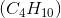?

Possible Answers: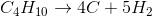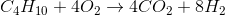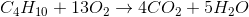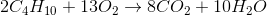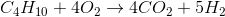Correct answer:Explanation:

Combustion is the chemical reaction of a hydrocarbon with molecular oxygen, and it always produces carbon dioxide and water. Knowing the reactants and products, the unbalanced equation must be: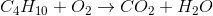We start by balancing the hydrogens. Since there are 10 on the left and only 2 on the right, we put a coefficient of 5 on water.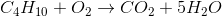Similarly, we balance carbons by putting a 4 on the carbon dioxide.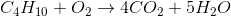To find the number of oxygens on the right, we multiply the 4 coefficient by the 2 subscript on O (which gets us 8 oxygens) and then add the 5 oxygens from the 5 water molecules to get a total of 13. The needed coefficient for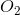on the left would then have to be 13/2.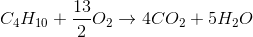Because fractional coefficients are not allowed, we mutiply every coefficient by 2 to find our final reaction: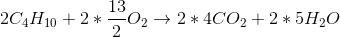### Example Question #1 : Chemical Equations

Determine whether or not solid aluminum reacts with aqueous zinc chloride. If it does, determine the balanced equation for the reaction.

Possible Answers:

No reaction occurs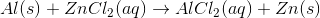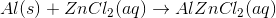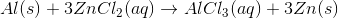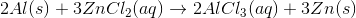Correct answer:Explanation:

When we check the activity series, it is fairly easy to see that aluminum metal is more reactive than zinc metal. So, in this case, the two metals undergo a redox reaction, where the aqueous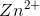is reduced to solid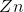, and the solid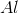is oxidized to aqueous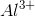. These charges are the common oxidation states for zinc and aluminum and should be memorized.

Becauseis the new species, it bonds with 3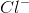ions. The unbalanced equation is: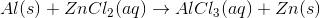We note that there are 2 chlorine atoms on the left and 3 chlorine atoms on the right. To balance, we use a 3 coefficient on the left and a 2 coefficient on the right. This gives a total of 6 chlorine atoms on eahc side.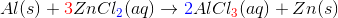However, now we have also increased the amounts of zinc and aluminum. We copy the necessary coefficients to balance those—2 for aluminum on the left, 3 for zinc on the right—and we are done:### Example Question #1 : Balancing Chemical Equations

_Fe2O3 + _HCl ⇌ _FeCl3 + _H2O

The Following question will be based on the unbalanced reaction above

For the reaction above to be balanced what coefficient should be in front of the compound HCl?

Possible Answers:

1

4

3

2

6

Correct answer:

6

Explanation:

Balancing reactions is best acheived by using a stepwise approach.  It is useful to work through each atom making sure it is present in a balanced fashion on both sides of the equation.  Starting with Fe, Fe2Ois the only molecule with Fe present on the left side of the equation and FeCl3 is the only molecule with Fe present on the right side of the equation.  Thus the molar ratio of Fe2Oto FeCl3 must be 1:2.  Moving on to O, the O on the left side of the equation exists as Fe2O3 and H2O on the right.  The molar ratio of Fe2Oto H2O is therefore 1:3.  Cl exists in HCl on the left and FeClon the right. Thus the molar ratio of HCl to FeCl3 must be 3:1. To ensure that these molar ratios are maintaned the balanced formula is then determined to be Fe2O3 + 6HCl -> 2FeCl3 + 3H2O

### Example Question #1 : Balancing Equations

Balance the following equation:

FeCl+ NOH→ Fe(OH)3 + NH4Cl

Possible Answers:

2 FeCl3 + 3 NOH5 → 2 Fe(OH)3 + 3 NH4Cl

FeCl3 + 3 NOH5 → Fe(OH)3 + 3 NH4Cl

3 FeCl3 + 3 NOH5 → 3 Fe(OH)3 + 3 NH4Cl

3 FeCl3 + 3 NOH5 → 2 Fe(OH)3 + 4 NH4Cl

2 FeCl3 + 4 NOH5 → Fe(OH)3 + 6 NH4Cl

Correct answer:

FeCl3 + 3 NOH5 → Fe(OH)3 + 3 NH4Cl

Explanation:

The first thing to do is to balance the Cl (3 on the left; one on the right) ← add 3 for NH4Cl

Now, there are 3 N on the right and only one on the left; add a 3 to the NOH5

Check to see that the Fe, H, and O balance, which they do

### Example Question #1 : Balancing Equations

After the following reaction is balanced, how many moles of H2O can 4 moles of C2Hproduce?

__C2H6(s) + ___O2(g) → ___H2O(l) + ___CO2(g)

Possible Answers:

9

6

3

12

8

Correct answer:

12

Explanation:

The reaction balances out to 2C2H6(s) + 7O2(g) → 6H2O(l) + 4CO2(g). For every  2 moles of  C2H6 you can produce 6 moles of H2O. Giving you a total production of 12 moles when you have 4 moles of C2H6.

### Example Question #1 : Balancing Equations

Consider the following reaction: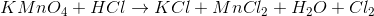When the equation is balanced, what will be the coefficient in front of HCl?

Possible Answers:

8

16

12

5

Correct answer:

16

Explanation:

When balancing equations, the goal is to make sure that the same atoms, in both type and amount, are on both the reactant and product side of the equation. A helpful approach is to write down the number of atoms already on both sides of the unbalanced equation. This way, you can predict which compounds need to be increased on which side in order to balance the equation. It also helps to balance oxygen and hydrogen last in the equation.

In this reaction, we can balance as follows.

Reactants: 1K, 1Mn, 1Cl, 4O, 1H

Products: 1K, 1Mn, 5Cl, 1O, 2H

So, we will need to increase H2O and HCl. The final balanced equation is written below.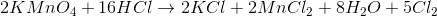### Example Question #1 : Balancing Equations

Balance the following chemical equation.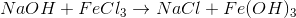Possible Answers: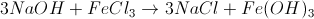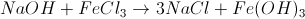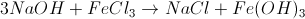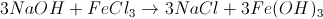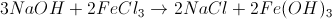Correct answer:Explanation:

To balance an equation, we need to make sure there is the same amount of elements to the left of the arrow as there is to the right. We also need all the charges to balance out. We notice right away that there are three chlorine atoms on the left, but only one on the right.(1Na, 1O, 1H, 1Fe, 3Cl : 1Na, 3O, 3H, 1Fe, 1Cl)

We can solve this by multiplying NaCl by three.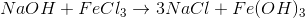(1Na, 1O, 1H, 1Fe, 3Cl : 3Na, 3O, 3H, 1Fe, 3Cl)

This causes us to have an imbalance of sodium, which we can correct by manipulating NaOH.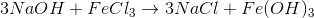(3Na, 3O, 3H, 1Fe, 3Cl : 3Na, 3O, 3H, 1Fe, 1Cl)

This is the final balanced equation. Note that it is usually easiest to manipulate oxygen and hydrogen last, since they are often involved in multiple molecules.

### Example Question #1 : Stoichiometry

Consider the following unbalanced equation for the combustion of propane,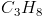: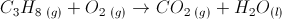If you were to combust one mole of propane, how many moles of water would you produce?

Possible Answers: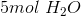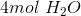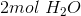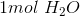Correct answer:Explanation:

Begin by balancing the equation. There are many ways to do this, but one method that is particularly useful is to assume that you have 1 mole of your hydrocarbon (propane), and balance the equation from there. It may be necessary to manipulate an equation further if you end up with fractions, but all you will need to do is multiply by an integer if that is the case.

First, we will balance the carbons. There are three carbons in propane, so we will make sure there are three carbons on the right side of the arrow as well: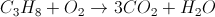This is not complete. We will next balance the hydrogens. There are eight hydrogens on the left side of the equation, so: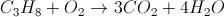The last step is to balance the oxygens on the left and right side of the equation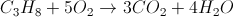Our equation is balanced and all coefficients are integers. If we begin with one mole of propane, we will produce four moles of water.

### Example Question #2 : Stoichiometry

Consider the following unbalanced equation: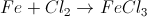How many grams of solid iron are needed to make 36.0g of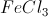? Assume that chlorine is in excess.

Possible Answers: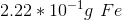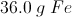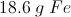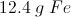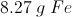Correct answer:Explanation:First, we will balance the equation: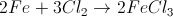Since chlorine is in excess, we know that the limiting reagent is iron.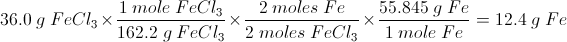### Example Question #11 : Balancing Chemical Equations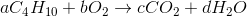Balance the above chemical reaction. What are the coefficients?

Possible Answers: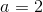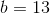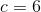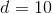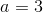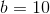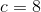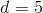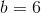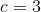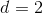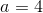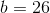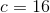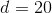Correct answer:Explanation:

First, balance the carbon atoms in the reactants and products. Next, balance the hydrogen atoms. Third, balnace the oxygen atoms. Since stoichiometric coefficients are written as integers, double everything to remove the decimal/fraction.

### All High School Chemistry Resources